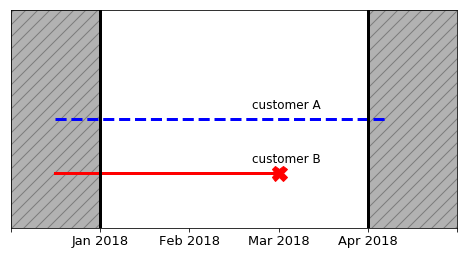# Introduction to Survival Analysis

## Introduction

Survival analysis is used to analyze or predict when an event is likely to happen. It originated from medical research, but its use has greatly expanded to many different fields. For instance:

## Censoring: why regression models cannot be used?

The real strength of Survival Analysis is its capacity to handle situations when the event has not happened yet. To illustrate this, let's take the example of two customers of a company and follow their active/churn status between January 2018 and April 2018:

• customer A started doing business prior to the time window, and as of April 2018, is still a client of the company.
• customer B also started doing business before January 2018, but churned in March 2018.Here, we have an explicit depiction of the event for customer B. However, we have no information about customer A, except that he/she hasn't churned yet at the end of the January 2018 to April 2018 time window. This situation is called censoring.

One might be tempted to use a regression model to predict when events are likely to happen. But to do that, one would need to disregard censored samples, which would result in a loss of important information. Fortunately, Survival models are able to take censoring into account and incorporate this uncertainty, so that instead of predicting the time of event, we are predicting the probability that an event happens at a particular time .

## Data format

We characterize survival analysis data-points with 3 elements: $\left( X_i, E_i, T_i \right)$, $\forall i$,

• $X_i$ is a p−dimensional feature vector.
• $E_i$ is the event indicator such that $E_i=1$, if an event happens and $E_i=0$ in case of censoring.
• $T_i = \min(t_i,c_i)$ is the observed time, with $t_i$ the actual event time and $c_i$ the time of censoring.

This configuration differs from regression modeling, where a data-point is defined by $\left( X_i, y_i \right)$ and $y_i$ is the target variable. This means that to fit a model, you will need to provide those 3 elements.

Let's look at the difference between a regression model fit and survival analysis one:

Modeling type code
Regression model using sklearn
from sklearn.linear_model import LinearRegression reg = LinearRegression() reg.fit(X=X_train, y=y_train) 
Survival analysis using pysurvival
from pysurvival.models.multi_task import LinearMultiTaskModelmtlr = LinearMultiTaskModel()  mtlr.fit(X=X_train, T=T_train, E=E_train)